SLVSAI0J October   2010  – May 2016

UNLESS OTHERWISE NOTED, this document contains PRODUCTION DATA.

1. Features
2. Applications
3. Description
4. Simplified Schematic
5. Revision History
6. Device Comparison Table
7. Pin Configuration and Functions
8. Specifications
9. Detailed Description
1. 9.1 Overview
2. 9.2 Functional Block Diagram
3. 9.3 Feature Description
4. 9.4 Device Functional Modes
10. 10Applications and Implementation
1. 10.1 Application Information
2. 10.2 Typical Application
11. 11Power Supply Recommendations
12. 12Layout
13. 13Device and Documentation Support
14. 14Mechanical, Packaging, and Orderable Information

• SIP|8

## 10 Applications and Implementation

NOTE

Information in the following applications sections is not part of the TI component specification, and TI does not warrant its accuracy or completeness. TI’s customers are responsible for determining suitability of components for their purposes. Customers should validate and test their design implementation to confirm system functionality.

### 10.1 Application Information

The TPS8267x devices are complete power supply modules, not needing further external devices. The devices are optimized to work best with the components populated. However application conditions might demand for different input and/or output capacitance values.

### 10.2 Typical Application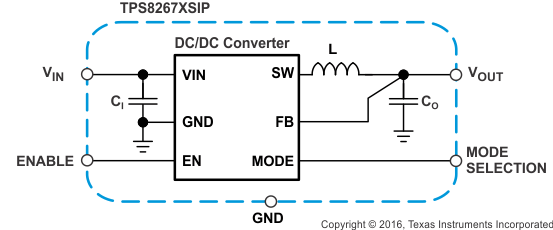Figure 10. MicroSIP Converter Module Schematic

#### 10.2.1 Design Requirements

For applications requiring additional input and/or output capacitance, the following procedures should be considered. For the maximum recommended values see Recommended Operating Conditions.

#### 10.2.2.1 Input Capacitor Selection

Because of the pulsating input current nature of the buck converter, a low ESR input capacitor is required to prevent large voltage transients that can cause misbehavior of the device or interference in other circuits in the system.

For most applications, the input capacitor that is integrated into the TPS8267x should be sufficient. If the application exhibits a noisy or erratic switching frequency, experiment with additional input ceramic capacitance to find a remedy.

The TPS8267x uses a tiny ceramic input capacitor. When a ceramic capacitor is combined with trace or cable inductance, such as from a wall adapter, a load step at the output can induce ringing at the VIN pin. This ringing can couple to the output and be mistaken as loop instability or can even damage the part. In this circumstance, additional "bulk" capacitance, such as electrolytic or tantalum, should be placed between the input of the converter and the power source lead to reduce ringing that can occur between the inductance of the power source leads and CI.

#### 10.2.2.2 Output Capacitor Selection

The advanced, fast-response, voltage mode, control scheme of the TPS8267x allows the use of a tiny ceramic output capacitor (CO). For most applications, the output capacitor integrated in the TPS8267x is sufficient.

At nominal load current, the device operates in PWM mode; the overall output voltage ripple is the sum of the voltage step that is caused by the output capacitor ESL and the ripple current that flows through the output capacitor impedance. At light loads, the output capacitor limits the output ripple voltage and provides holdup during large load transitions.

The TPS8267x is designed as a Point-Of-Load (POL) regulator, to operate stand-alone without requiring any additional capacitance. Adding a 2.2μF ceramic output capacitor (X7R or X5R dielectric) generally works from a converter stability point of view, but does not necessarily help to minimize the output ripple voltage.

For best operation (i.e. optimum efficiency over the entire load current range, proper PFM/PWM auto transition), the TPS8267xSIP requires a minimum output ripple voltage in PFM mode. The typical output voltage ripple is ca. 1% of the nominal output voltage VO. The PFM pulses are time controlled resulting in a PFM output voltage ripple and PFM frequency that depends (first order) on the capacitance seen at the MicroSiPTM DC/DC converter's output.

In applications requiring additional output bypass capacitors located close to the load, care should be taken to ensure proper operation. If the converter exhibits marginal stability or erratic switching frequency, experiment with additional low value series resistance (e.g. 50 to 100mΩ) in the output path to find a remedy.

Because the damping factor in the output path is directly related to several resistive parameters (e.g. inductor DCR, power-stage rDS(on), PWB DC resistance, load switches rDS(on) …) that are temperature dependant, the converter small and large signal behavior must be checked over the input voltage range, load current range and temperature range.

The easiest sanity test is to evaluate, directly at the converter’s output, the following aspects:

• PFM/PWM efficiency
• PFM/PWM and forced PWM load transient response

During the recovery time from a load transient, the output voltage can be monitored for settling time, overshoot or ringing that helps judge the converter’s stability. Without any ringing, the loop has usually more than 45° of phase margin.

#### 10.2.3 Application Curves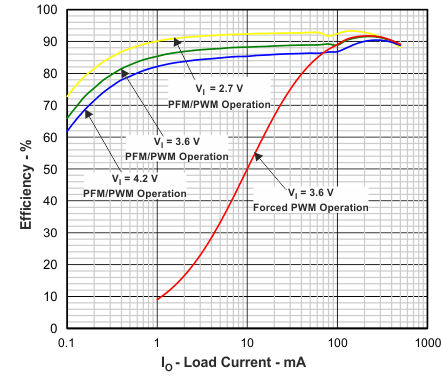VO = 1.95V
Figure 11. Efficiency vs. Load Current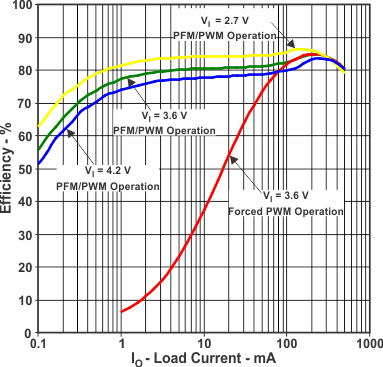VO = 1.2 V
Figure 13. Efficiency vs. Load Current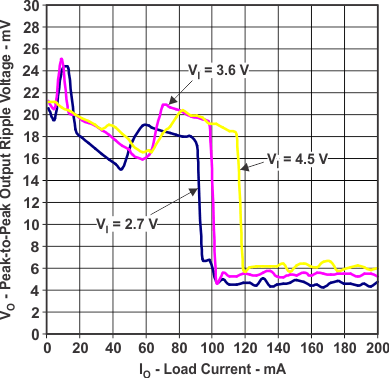VO = 1.8 V PFM/PWM Operation (TPS82671)
Figure 15. Peak-to-Peak Output Ripple Voltage vs. Load Current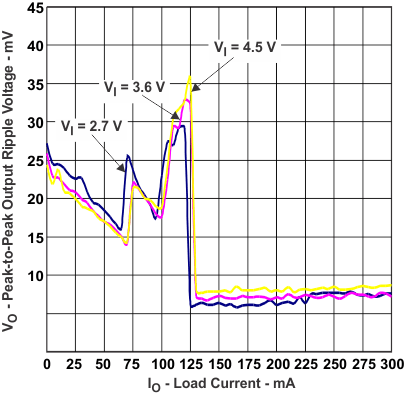VO = 1.2 V (TPS82671)
Figure 17. Peak-to-Peak Output Ripple Voltage vs. Load Current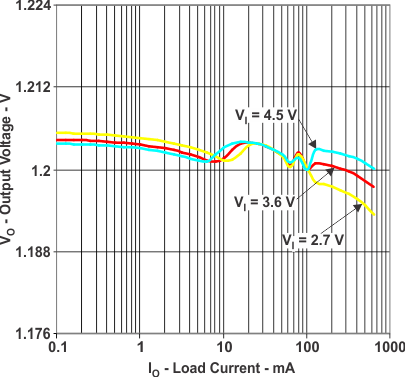VO = 1.2 V (TPS82675)
Figure 19. DC Output Voltage vs. Load Current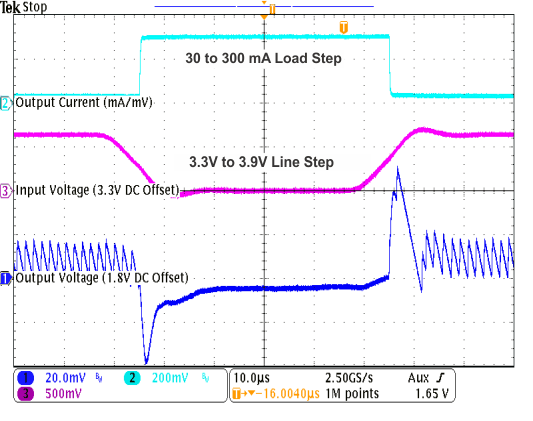VO = 1.8 V MODE = Low (TPS82671)
Figure 21. Combined Line/Load Transient Response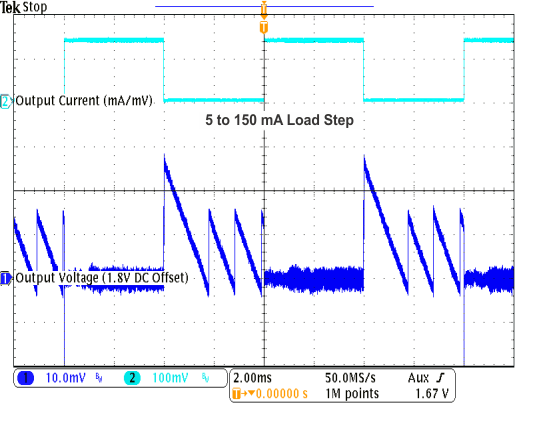VO = 1.8 V VI = 3.6 V MODE = Low (TPS82671)
Figure 23. Load Transient Response in PFM/PWM Operation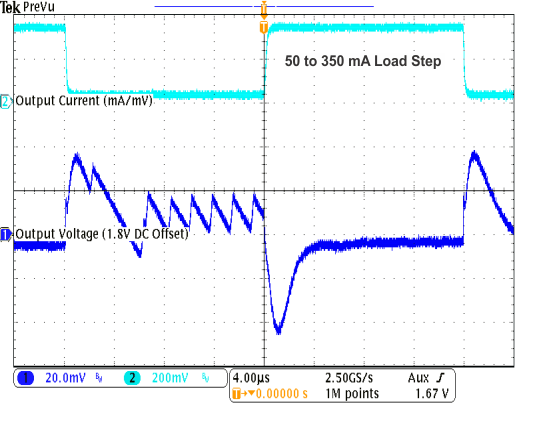VO = 1.8 V VI = 2.7 V MODE = Low (TPS82671)
Figure 25. Load Transient Response in PFM/PWM Operation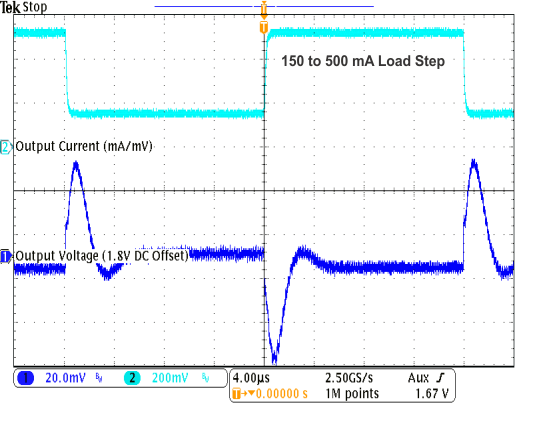VO = 1.8 V VI = 3.6 V MODE = Low (TPS82671)
Figure 27. Load Transient Response in PFM/PWM Operation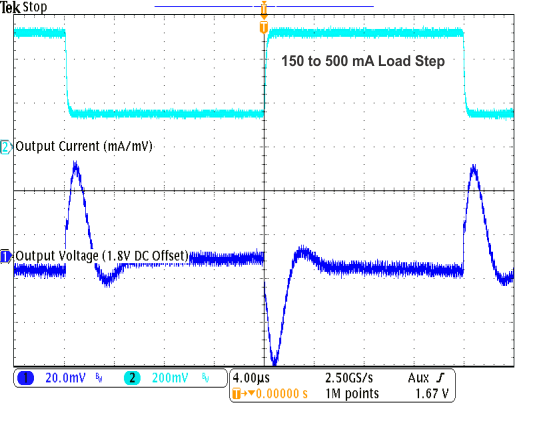VO = 1.8 V VI = 4.5 V MODE = Low (TPS82671)
Figure 29. Load Transient Response in PFM/PWM Operation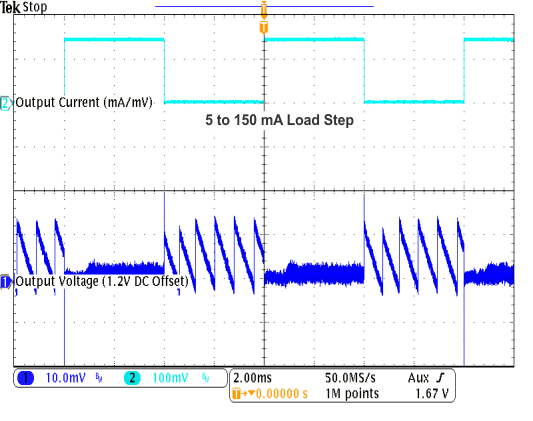VO = 1.2 V VI = 3.6 V MODE = Low
Figure 31. Load Transient Response in PFM/PWM Operation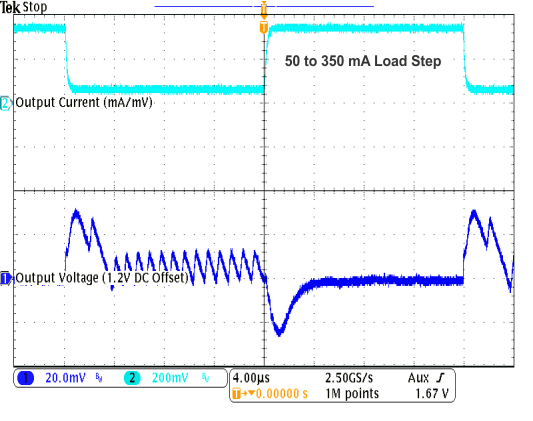VO = 1.2 V VI = 2.7 V MODE = Low
Figure 33. Load Transient Response in PFM/PWM Operation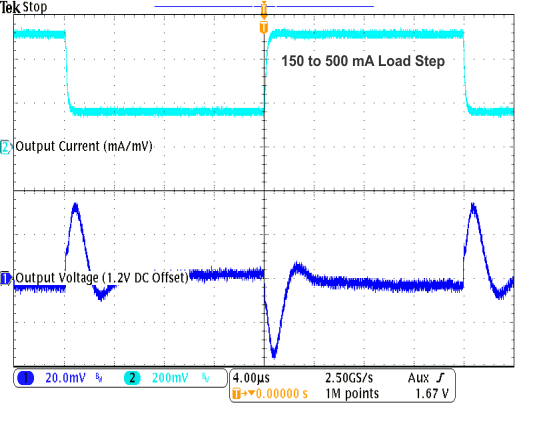VO = 1.2 V VI = 3.6 V MODE = Low
Figure 35. Load Transient Response in PFM/PWM Operation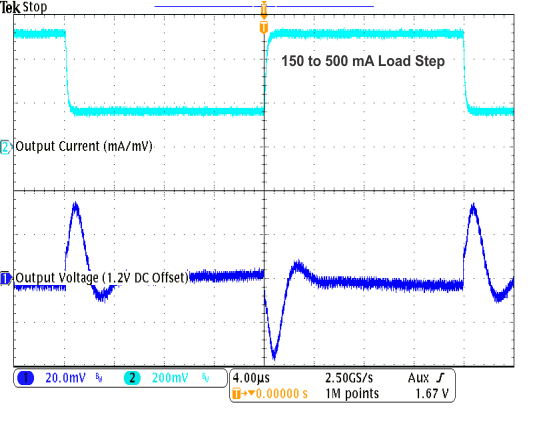VO = 1.2 V VI = 4.5 V MODE = Low (TPS82671)
Figure 37. Load Transient Response in PFM/PWM OperationA.
 VO = 1.8 V (TPS82671)
Figure 39. PFM/PWM Boundaries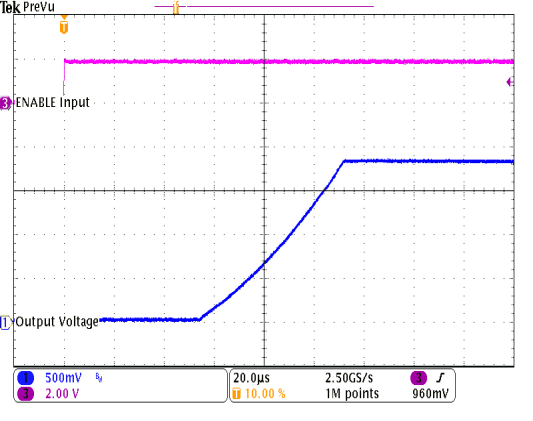VO = 1.8 V VI = 3.6 V IO = 0 mA MODE = Low
Figure 41. Start-Up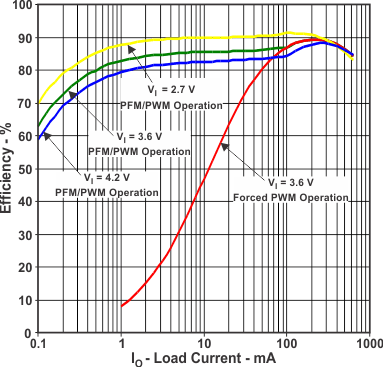VO = 1.8 V
Figure 12. Efficiency vs. Load Current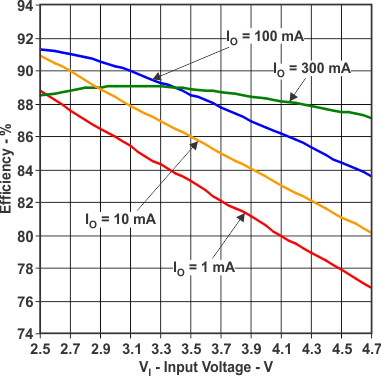VO = 1.8 V PFM/PWM Operation
Figure 14. Efficiency vs. Input Voltage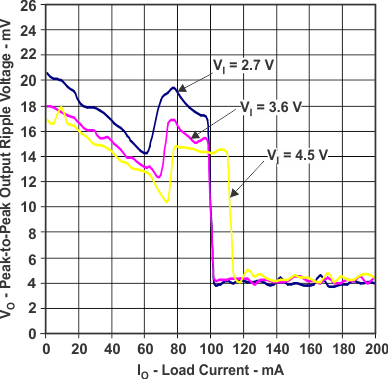VO = 1.2 V PFM/PWM Operation (TPS82675)
Figure 16. Peak-to-Peak Output Ripple Voltage vs. Load Current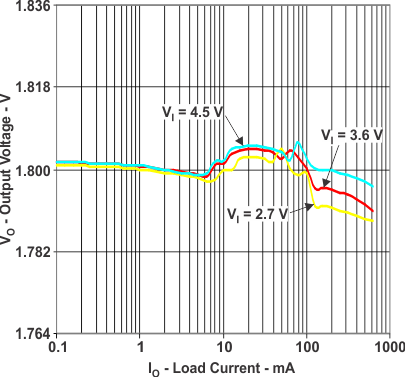VO = 1.8 V PFM/PWM Operation (TPS82671)
Figure 18. DC Output Voltage vs. Load Current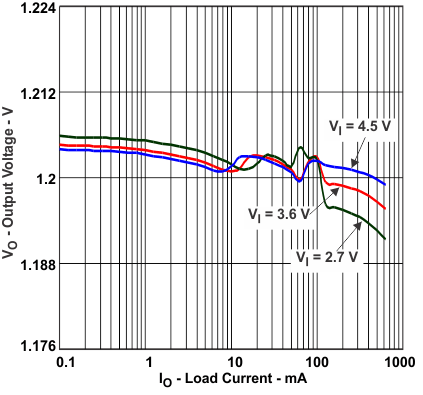VO = 1.2 V PFM/PWM Operation (TPS82677)
Figure 20. DC Output Voltage vs. Load Current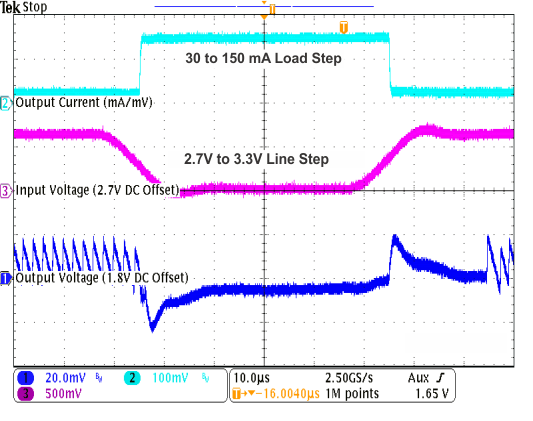VO = 1.8 V MODE = Low (TPS82671)
Figure 22. Combined Line/Load Transient Response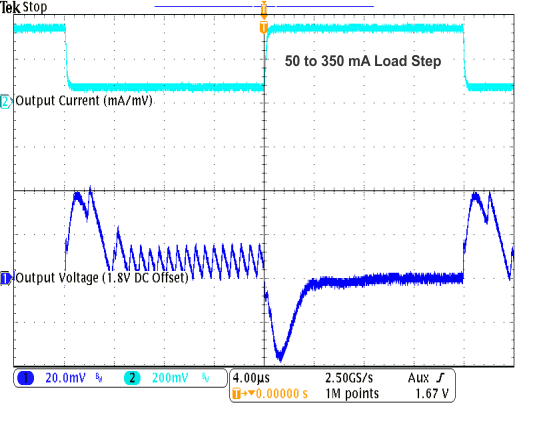VO = 1.8 V VI = 3.6 V MODE = Low (TPS82671)
Figure 24. Load Transient Response in PFM/PWM Operation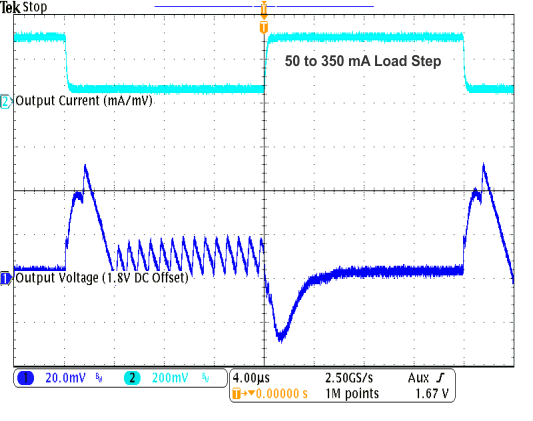VO = 1.8 V VI = 4.5 V MODE = Low (TPS82671)
Figure 26. Load Transient Response in PFM/PWM Operation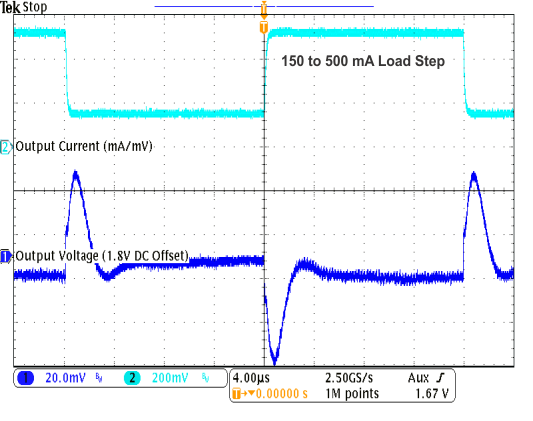VO = 1.8 V VI = 2.7 V MODE = Low (TPS82671)
Figure 28. Load Transient Response in PFM/PWM Operation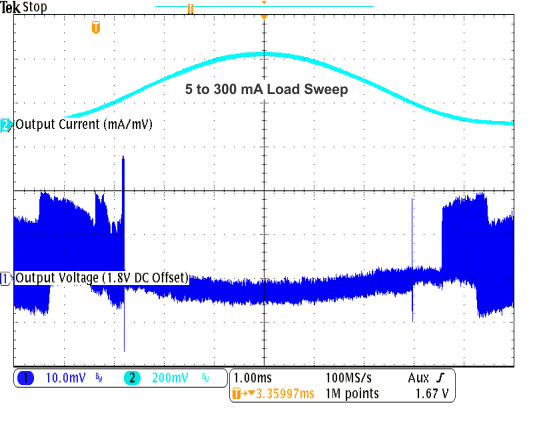VO = 1.8 V VI = 3.6 V MODE = Low (TPS82671)
Figure 30. AC Load Transient Response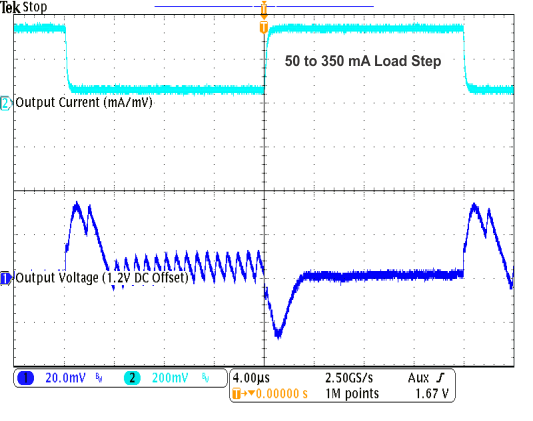VO = 1.2 V VI = 3.6 V MODE = Low
Figure 32. Load Transient Response in PFM/PWM Operation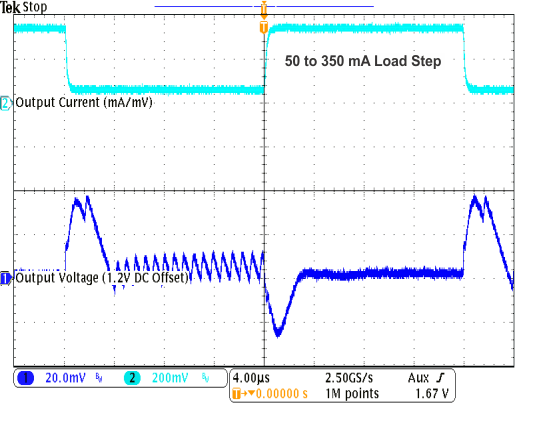VO = 1.2 V VI = 4.5 V MODE = Low
Figure 34. Load Transient Response in PFM/PWM Operation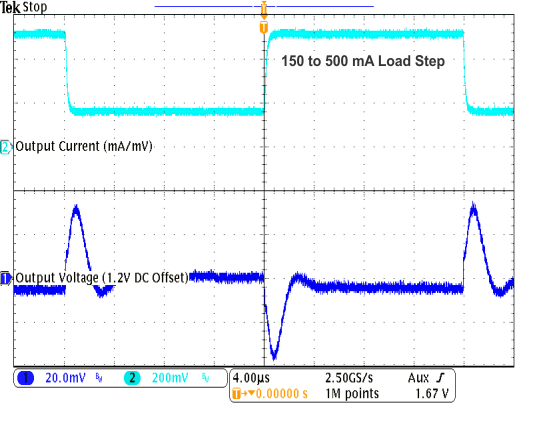VO = 1.2 V VI = 2.7 V MODE = Low
Figure 36. Load Transient Response in PFM/PWM Operation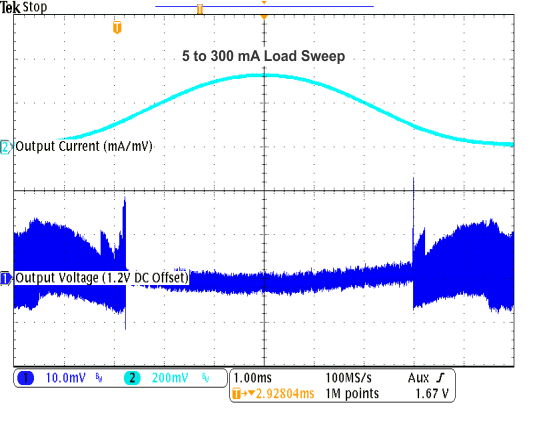VO = 1.2 V VI = 3.6 V MODE = Low (TPS82671)
Figure 38. AC Load Transient Response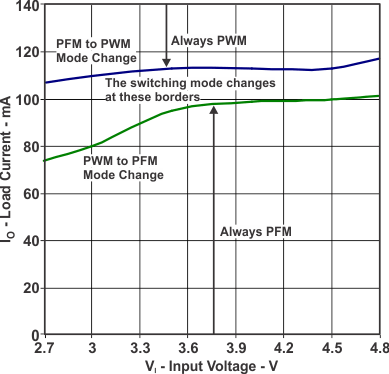VO = 1.2 V (TPS82674)
Figure 40. PFM/PWM Boundaries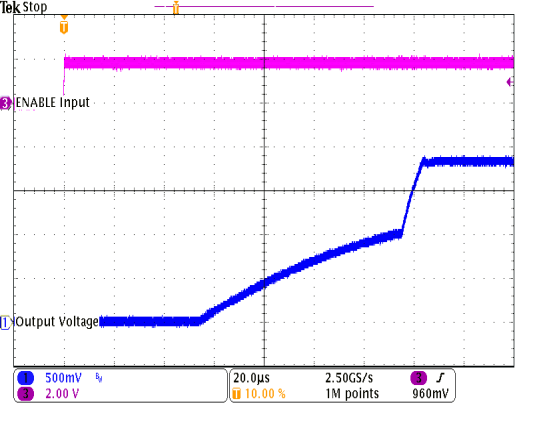VO = 1.8 V VI = 3.6 V (TPS82671) RL = 100 Ω MODE = Low
Figure 42. Start-Up